# C++语言与C的不同之处

##### 头文件

C语言的引用头文件的方式是include名字`.h`的方式进行引用，而C++去掉了.h，但是又是完全兼容C语言的。在库前面添加一个c来表明这个库是来自于C语言的。

``````//C语言的方法：带.h的方式进行include
#include<stdio.h>
#include<math.h>

//C++的方法，直接引用即可
#include<cstdio>
#include<cmath>
``````

``````#include<iostream> //输入输出流所包含的头文件

using namespace std;  //C++标准库中的类和函数是在命名空间std中声明的

``````

c++完全兼容c，因此c语言的标准库在c++中也是可以使用的，所以为了区分c++程序需要使用`using namespace std;`重新定义c++的标准库，不至于与c的标准库混淆。

##### 输入输出函数

C语言输出是格式化输出，必须指定输出类型。

``````int n; //定义n为整形
//C语言的输入输出（需要指定类型，如下指定为整形）
scanf("%d",&n);
printf("%d",n);
``````

C++输出采用流的思想输出，无需指明数据类型，注意必须引入`iostream`库函数时才可以使用。

``````//C++语言的输入输出（不需要指定类型，会根据n进行自主的判定）
cin>>n;
cout<<n;
``````

`<algorithm>、<deque>、<functional>、<iterator>、<vector>、<list>、<map>、<memory>、<numeric>、<queue>、<set>、<stack>和<utility>`

##### 串

``````int main(){

/* int n; cin>>n; cout<<n; */

char chr = {
'h','e','l','l','o'};
int i;
for(i=0;i<5;i++){

printf("%c",chr[i]);
}
char *p = "hello";
int i;
for(i=0;i<5;i++){

printf("%c",p[i]);
}
return 0;
}
``````
``````#include<iostream>
using namespace std;

int main(){

string str = "hello";
cout<<str;
return 0;
}
``````
##### 引用

①一个变量可以有多个别名。

②引用必须被初始化。

③引用在初始化时只能引用一次，不能更改为引用其他变量。

``````int a = 10;
int &b = a;
``````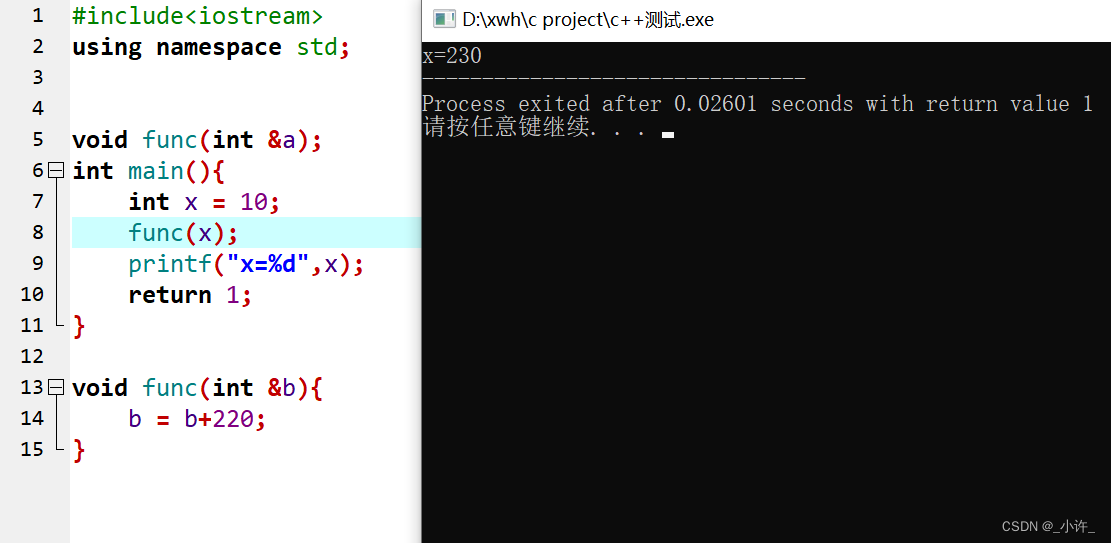• 普通变量作为返回值
``````#include<iostream>
using namespace std;

int func(int &a);
int main(){

int x = 1000;
int a = func(x);
printf("%d",a);
}

//普通返回值
int func(int &b){

return b+1000;       //值传递产生新的地址空间（局部变量赋值新地址后销毁）
}
``````

• 指针返回值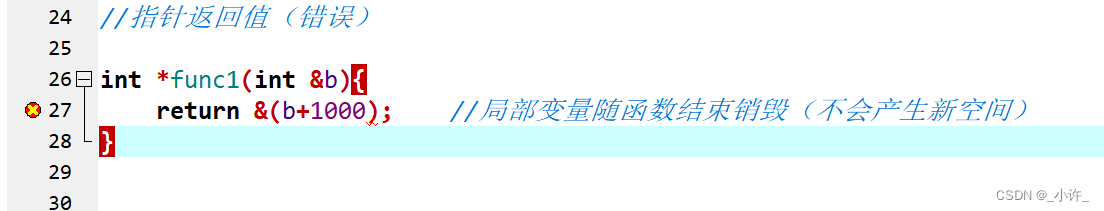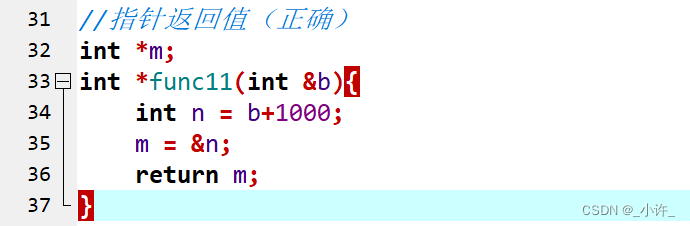• 作为返回值的引用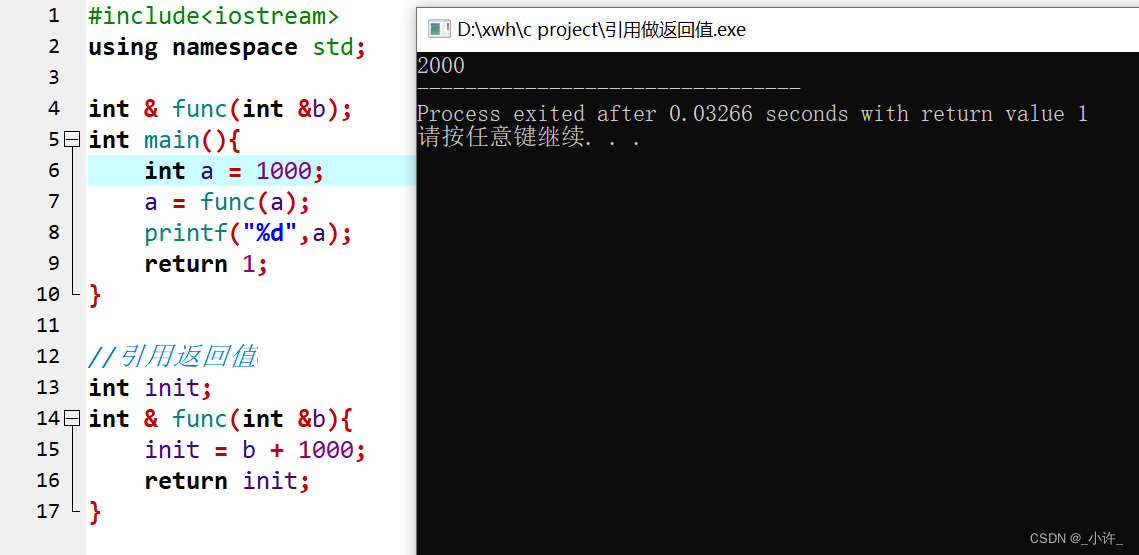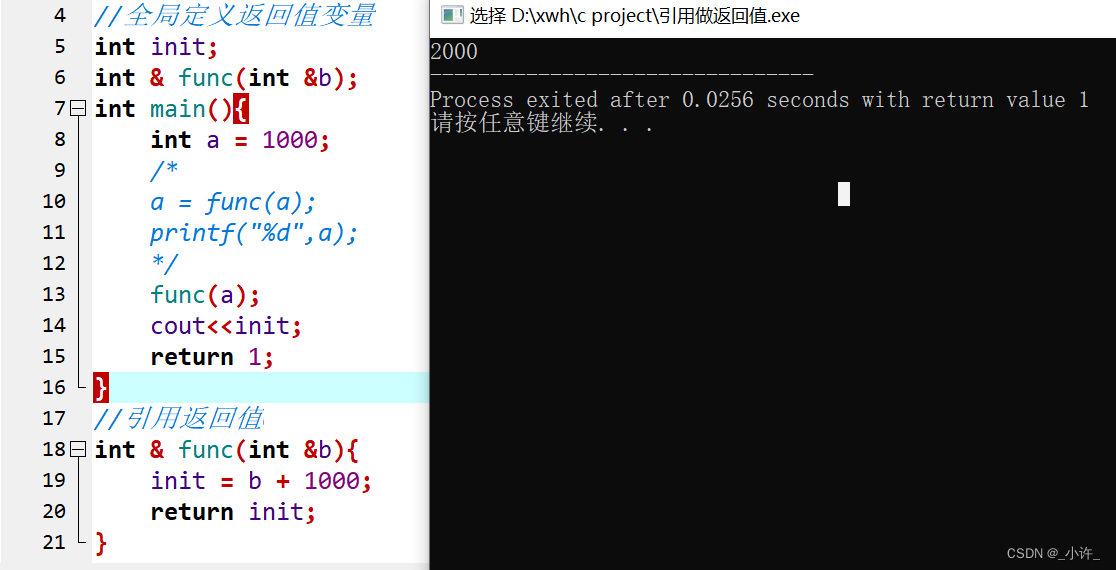``````#include<iostream>
using namespace std;

//全局定义返回值变量
int init;
int & func(int &b);
int main(){

int a = 1000;
/* a = func(a); printf("%d",a); */
func(a);
cout<<init;
return 1;
}
//引用返回值
int & func(int &b){

init = b + 1000;
return init;
}
``````

##### 类

c++添加看新的特性种类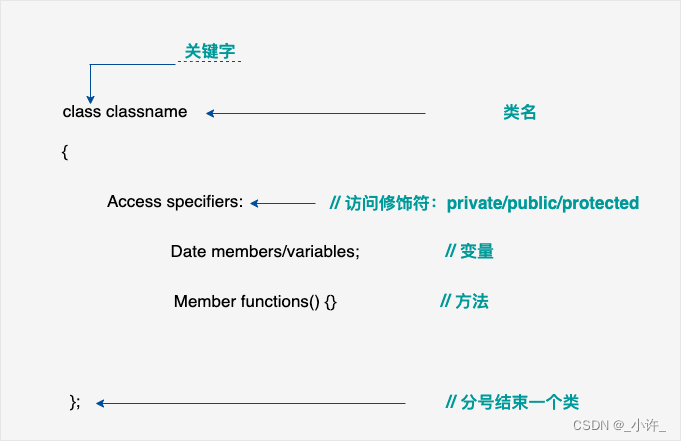##### 异常处理

c++提供全新的异常处理机制，处理程序执行期间出现的各种问题。C++ 异常处理涉及到三个关键字：`try、catch、throw`

1. throw：当出现问题时，程序会抛出异常。这是使用 throw 关键字完成的。
2. catch：通过异常处理程序捕获要处理问题的异常。 catch 关键字用于捕获异常。
3. try：try块中的代码标识将激活的特定异常。它后面通常跟随一个或多个 catch 块。
``````try
{

// 保护代码
}catch( ExceptionName e1 )
{

// catch 块
}catch( ExceptionName e2 )
{

// catch 块
}catch( ExceptionName eN )
{

// catch 块
}
``````

``````double division(int a, int b)
{

if( b == 0 )
{

throw "Division by zero condition!";
}
return (a/b);
}
``````

C++ 标准异常

##### 动态内存分配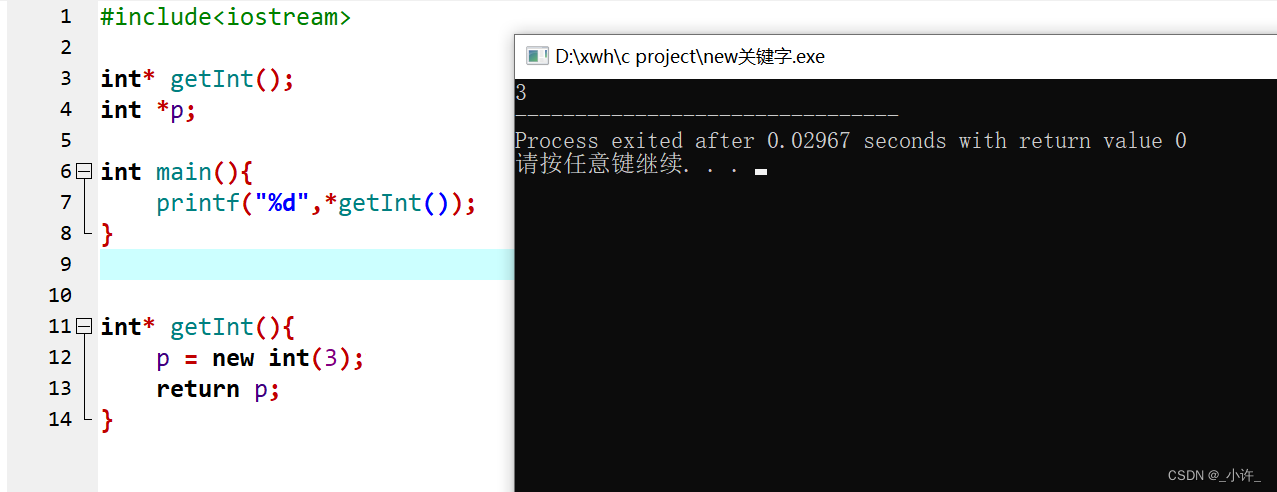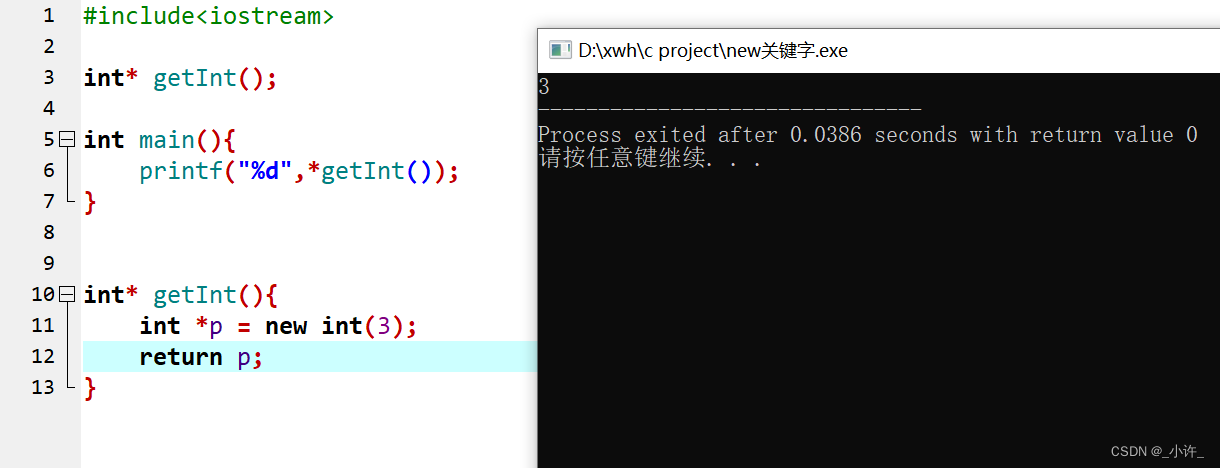##### C++ 命名空间

C++命名空间

toString 方法在 test.h 中定义。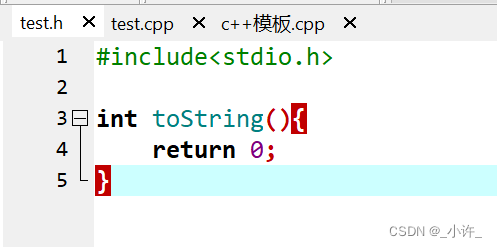test.c中引入test.h并再次定义toString方法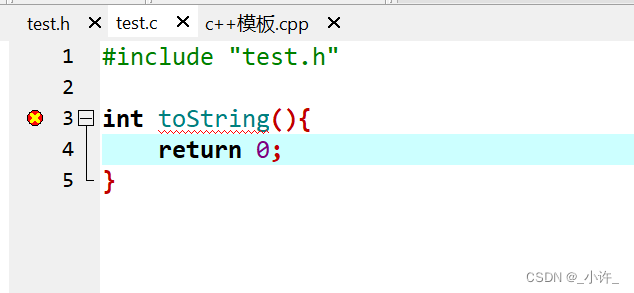• 定义

c++中通过`namespace space_name`定义命名空间，方法和变量都定义在命名空间中，如下：

``````#include<iostream>

namespace myspace{

//std是默认导入的标准库 using namespace std
int toString(int str){

cout<<str;
}
}
``````

• 转移

``````space_name :: code;
``````

1. 头文件test.hpp中定义命名空间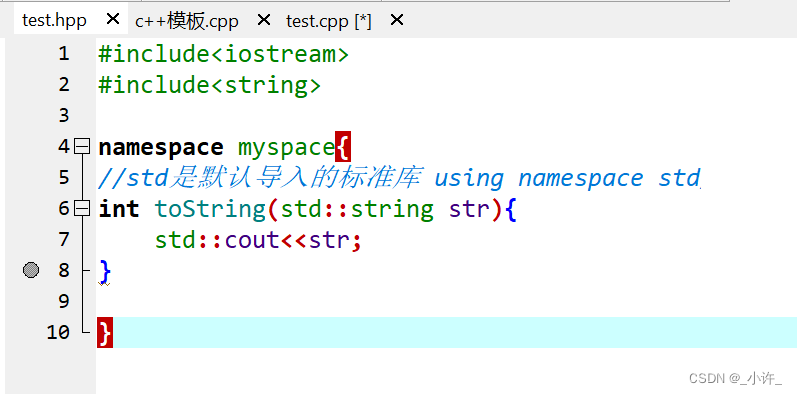2. 引入头文件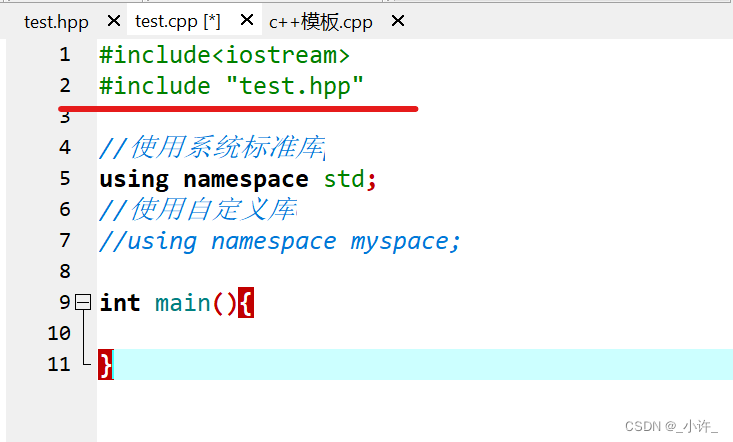1. 命名空间名称调用方法或变量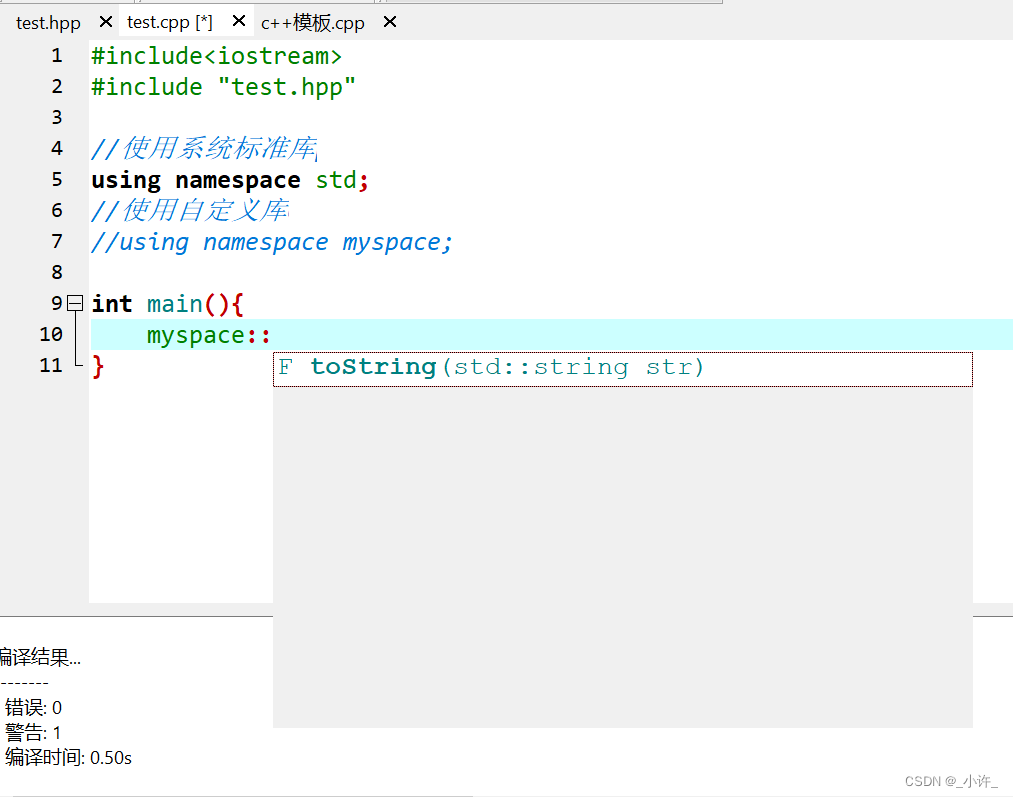``````//如必要的系统标准库的引入
using namespace std;
//自定义的引入
using namespace myspace;
``````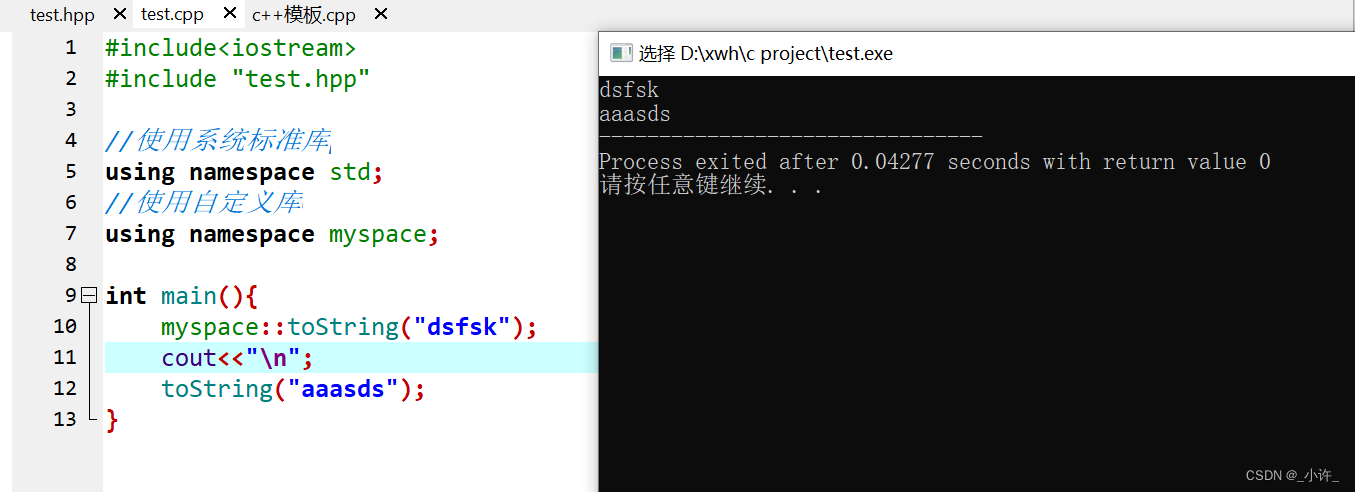##### 泛型

1. 了解泛型
``````#include<iostream>
using namespace std;
class IntArray{

private : int item;
private : int cacur = 0;
public :
void Push(int x){

item[cacur] = x;
cacur ++;
}

int Pop(){

cacur --;
int e = item[cacur];
return e;
}
};

int main(){

IntArray arr;
for(int i=0;i<=5;i++){

//TODO
arr.Push(i+1);
}

for(int i=0;i<=5;i++){

//TODO
int e = arr.Pop();
cout<<e;
}
}
``````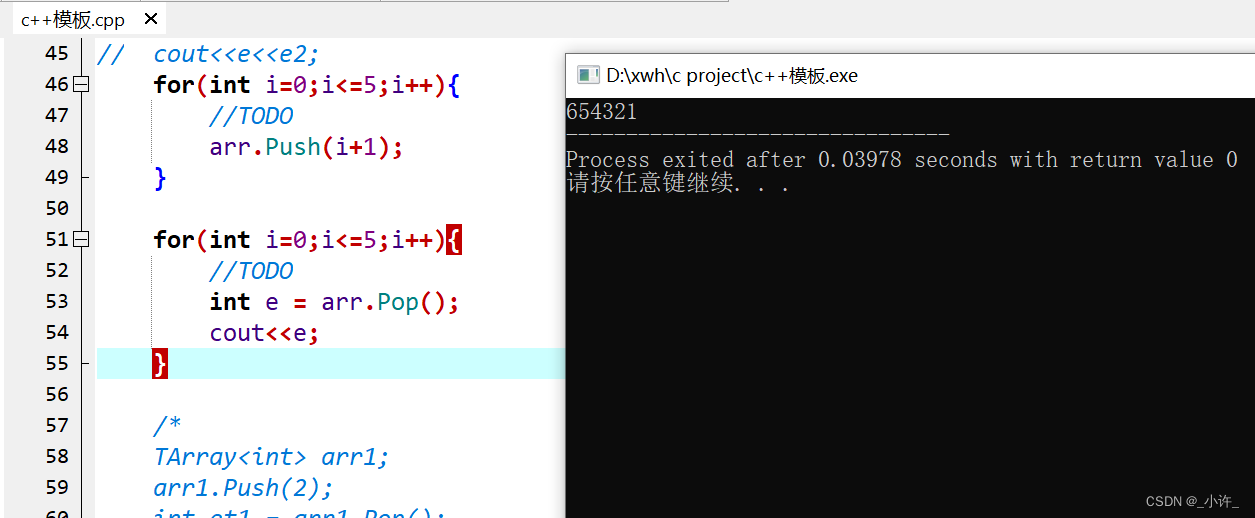1. 使用泛型
``````#include<iostream>
using namespace std;

template <typename T>
T Max(T a,T b){

return a>b?a:b;
}

int main(){

cout<<Max(6,5);
cout<<Max(6.5,5.4);
cout<<Max('c','a');
}
``````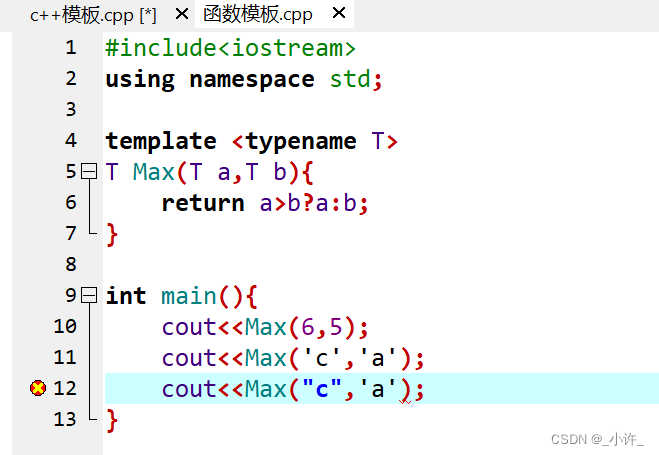``````template <typename T>
``````
``````template <typename T>
T Max(T a,T b){

return a>b?a:b;
}
``````

``````template <class T>
``````
``````template <class T>
class TArray{

private : T item;
private : int cacur = 0;
public :
void Push(T x){

item[cacur] = x;
cacur ++;
}

T Pop(){

cacur --;
T e = item[cacur];
return e;
}
};
``````

``````int main{

TArray<int> arr1;
//存储int
arr1.Push(2);
//存储float
arr1.Push(4.7);
//存储char
arr1.Push('a');

int et1 = arr1.Pop();
int et2 = arr.Pop();
int et3 = arr1.Pop();
cout<<et1<<"-"<<et2<<"-"<<et3;
return 0;
}
``````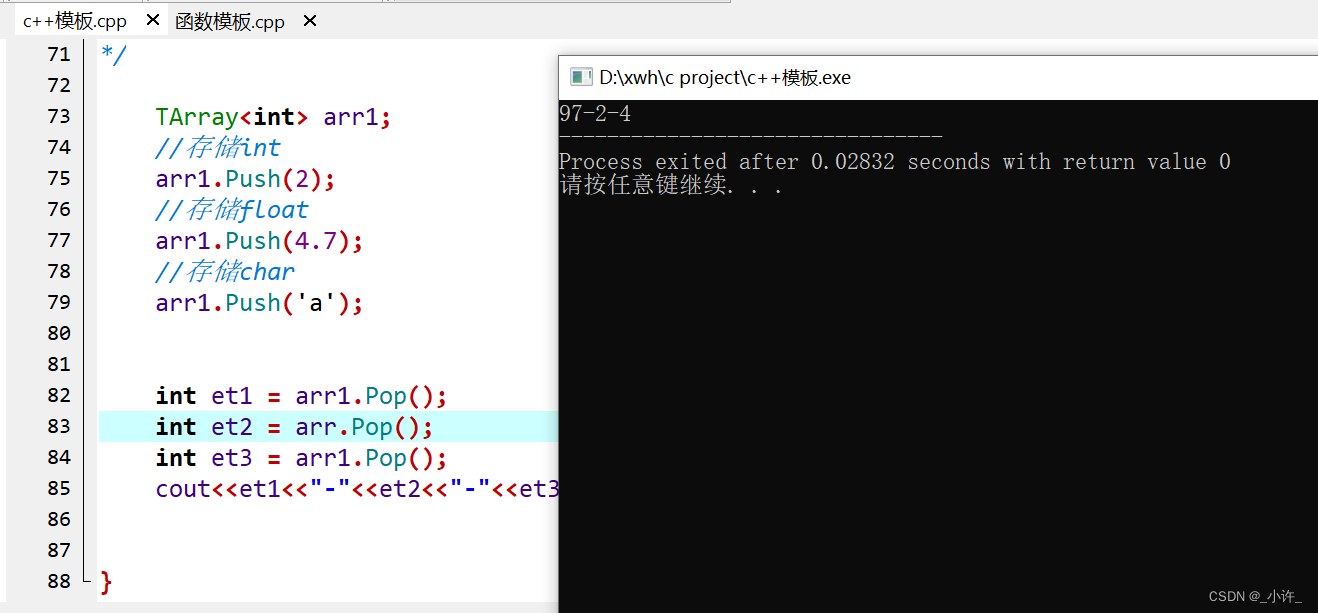``````TArray<int> arr;
``````
``````TArray<int> arr1;
//存储int
arr1.Push(2);
//存储float
arr1.Push(4);
//存储char
arr1.Push(5);

int et1 = arr1.Pop();
int et2 = arr.Pop();
char et3 = arr1.Pop();
cout<<et1<<"-"<<et2<<"-"<<et3;
``````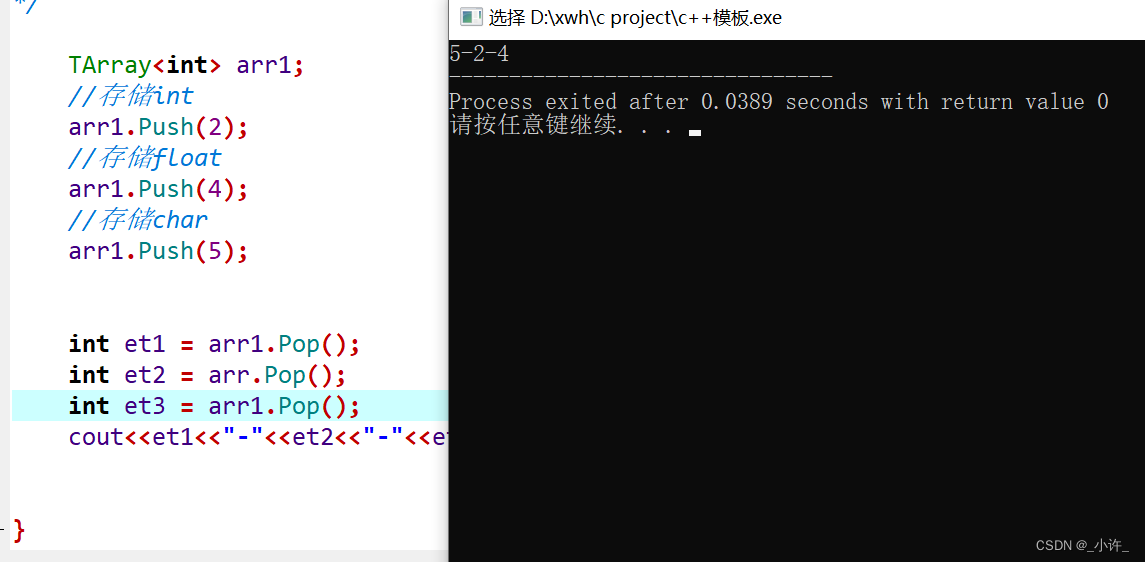·

. . .

## 相关推荐

#### js + css实现左侧悬浮导航栏

<html> <head> <title>浮在页面上的导航菜单</title> <meta content="text/html; charset=gb2312" http-equiv="Content-Type"> <mce:style><!-- bo

30天前
29天前
25天前
25天前
25天前
25天前
22天前
17天前
17天前
17天前

30天前
29天前
25天前
25天前
25天前
25天前
25天前
25天前
25天前
17天前